#### 期刊菜单

The Analytical Solution and Application of a Coupled Shallow Water Wave Equation
DOI: 10.12677/AAM.2022.115268, PDF, HTML, XML, 下载: 41  浏览: 63  国家自然科学基金支持

Abstract: In this paper, solutions of a set of coupled nonlinear shallow water wave equations are solved based on the analytical method, and the results are discussed. The behavior of the equation solution un-der different parameters is studied. We concluded that the dispersion coefficient can significantly affect the dynamics of the shallow water wave. Based on the equations given in this paper, some ex-tended applications under special parameters are discussed. The result presented in this paper may give insights to the relevant studies of the shallow water wave equation.

1. 引言

${\eta }_{t}+{\left(u\eta \right)}_{x}+\alpha {u}_{xxx}-\beta {\eta }_{xx}=0,$ (1a)

${u}_{t}+{\eta }_{x}+u{u}_{x}+\beta {u}_{xx}=0,$ (1b)

2. 方法介绍

$P\left(u,{u}_{t},{u}_{x},{u}_{xx},{u}_{tt},{u}_{xt},\cdots \right)=0.$ (2)

$\xi =kx-\omega t,$ (3)

$P\left(u,{u}^{\prime },{u}^{″},\cdots \right)=0.$ (4)

$u\left(\xi \right)=\underset{n=0}{\overset{N}{\sum }}{a}_{n}{Q}^{n}\left(\xi \right),$ (5)

$Q\left(\xi \right)=\frac{1}{1+q{A}^{\xi }}.$ (6)

${Q}^{\prime }=\left({Q}^{2}-Q\right)\mathrm{ln}A.$ (7)

$\text{Deg}\left[{u}^{m}\left(\xi \right){\left(\frac{{\text{d}}^{s}{u}^{r}\left(\xi \right)}{\text{d}{\xi }^{s}}\right)}^{q}\right]=np+q\left(mr+s\right).$ (8)

$P\left(Q\left(\xi \right)\right)=0.$ (9)

3. 浅水波的行波解

$-\omega {u}^{\prime }+ku{u}^{\prime }+\beta {k}^{2}{u}^{″}+k{\eta }^{\prime }=0,$ (10a)

$-\omega {\eta }^{\prime }-\beta {k}^{2}{\eta }^{″}+k{\left(u\eta \right)}^{\prime }+\alpha {k}^{3}{u}^{‴}=0,$ (10b)

$-\omega u+\frac{1}{2}k{u}^{2}+\beta {k}^{2}{u}^{\prime }+k\eta +C=0.$ (11)

$\eta =\frac{\omega }{k}u-\frac{1}{2}{u}^{2}-\beta k{u}^{\prime }+C.$ (12)

$\left(\alpha +{\beta }^{2}\right){k}^{3}{u}^{‴}-\frac{3}{2}k{u}^{2}{u}^{\prime }+3\omega u{u}^{\prime }+\left(Ck-\frac{{\omega }^{2}}{k}\right){u}^{\prime }=0.$ (13)

$6{k}^{3}\left(\alpha +{\beta }^{2}\right){\mathrm{ln}}^{3}A{a}_{1}-\frac{3}{2}k\mathrm{ln}A{a}_{1}=0,$ (14a)

$\frac{3}{2}k\mathrm{ln}A{a}_{1}^{3}+3\left(\omega -k{a}_{0}\right)\mathrm{ln}A{a}_{1}^{2}-12\left(\alpha +{\beta }^{2}\right){k}^{3}{\mathrm{ln}}^{3}A{a}_{1}=0,$ (14b)

$3\left(k{a}_{0}-\omega \right)\mathrm{ln}A{a}_{1}^{2}+7\left(\alpha +{\beta }^{2}\right){k}^{3}{\mathrm{ln}}^{3}A{a}_{1}+3\left(\omega -\frac{k}{2}{a}_{0}^{2}\right){a}_{1}+\left(Ck-\frac{{\omega }^{2}}{k}\right)\mathrm{ln}A{a}_{1}=0,$ (14c)

$\left(\alpha +{\beta }^{2}\right){k}^{3}\mathrm{ln}A{a}_{1}-3\left(\omega -\frac{k}{2}{a}_{0}^{2}\right)\mathrm{ln}A{a}_{1}+\left(Ck-\frac{{\omega }^{2}}{k}\right)\mathrm{ln}A{a}_{1}=0.$ (14d)

${a}_{0}=\frac{-2\left(\alpha +{\beta }^{2}\right)k\mathrm{ln}A+{R}_{l}{R}_{s}}{{R}_{s}},$ (15a)

${a}_{1}=k\mathrm{ln}A{R}_{s},$ (15b)

$k=k,$ (15c)

$\omega =k{R}_{l}.$ (15d)

${u}_{1}=\frac{\sqrt{\left(\alpha +2{\beta }^{2}\right)\left[\left(\alpha +{\beta }^{2}\right){k}^{2}{\mathrm{ln}}^{2}A-2C\right]}}{\sqrt{\alpha +{\beta }^{2}}}-\frac{\left(\alpha +{\beta }^{2}\right)k\mathrm{ln}A}{\sqrt{\alpha +{\beta }^{2}}}+\frac{2\sqrt{\alpha +{\beta }^{2}}k\mathrm{ln}A}{1+q{A}^{\xi }},$ (16a)

${\eta }_{1}=\frac{2\left(\alpha +{\beta }^{2}+\beta \sqrt{\alpha +{\beta }^{2}}\right){k}^{2}q{A}^{\xi }{\mathrm{ln}}^{2}A}{{\left(1+q{A}^{\xi }\right)}^{2}}.$ (16b)

${u}_{2}=\frac{-\sqrt{\left(\alpha +2{\beta }^{2}\right)\left[\left(\alpha +{\beta }^{2}\right){k}^{2}{\mathrm{ln}}^{2}A-2C\right]}}{\sqrt{\alpha +{\beta }^{2}}}+\frac{\left(\alpha +{\beta }^{2}\right)k\mathrm{ln}A}{\sqrt{\alpha +{\beta }^{2}}}-\frac{2\sqrt{\alpha +{\beta }^{2}}k\mathrm{ln}A}{1+q{A}^{\xi }},$ (17a)

${\eta }_{2}=\frac{2\left(\alpha +{\beta }^{2}-\beta \sqrt{\alpha +{\beta }^{2}}\right){k}^{2}q{A}^{\xi }{\mathrm{ln}}^{2}A}{{\left(1+q{A}^{\xi }\right)}^{2}}.$ (17b)

${u}_{3}=\frac{-\sqrt{\left(\alpha +2{\beta }^{2}\right)\left[\left(\alpha +{\beta }^{2}\right){k}^{2}{\mathrm{ln}}^{2}A-2C\right]}}{\sqrt{\alpha +{\beta }^{2}}}-\frac{\left(\alpha +{\beta }^{2}\right)k\mathrm{ln}A}{\sqrt{\alpha +{\beta }^{2}}}+\frac{2\sqrt{\alpha +{\beta }^{2}}k\mathrm{ln}A}{1+q{A}^{\xi }},$ (18a)

${\eta }_{3}=\frac{2\left(\alpha +{\beta }^{2}+\beta \sqrt{\alpha +{\beta }^{2}}\right){k}^{2}q{A}^{\xi }{\mathrm{ln}}^{2}A}{{\left(1+q{A}^{\xi }\right)}^{2}}.$ (18b)

${u}_{4}=\frac{-\sqrt{\left(\alpha +2{\beta }^{2}\right)\left[\left(\alpha +{\beta }^{2}\right){k}^{2}{\mathrm{ln}}^{2}A-2C\right]}}{\sqrt{\alpha +{\beta }^{2}}}+\frac{\left(\alpha +{\beta }^{2}\right)k\mathrm{ln}A}{\sqrt{\alpha +{\beta }^{2}}}-\frac{2\sqrt{\alpha +{\beta }^{2}}k\mathrm{ln}A}{1+q{A}^{\xi }},$ (19a)

${\eta }_{4}=\frac{2\left(\alpha +{\beta }^{2}-\beta \sqrt{\alpha +{\beta }^{2}}\right){k}^{2}q{A}^{\xi }{\mathrm{ln}}^{2}A}{{\left(1+q{A}^{\xi }\right)}^{2}}.$ (19b)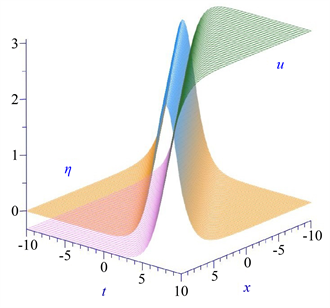(a)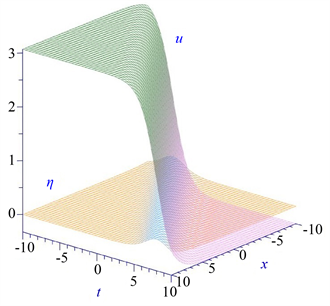(b)

Figure 1. The evolutions of velocity field $u\left(x,t\right)$ and $\eta \left(x,t\right)$ for solution groups 1(a) and 2(b). Here $\alpha =2$, $\beta =2$, $A=2$, $q=2$, $C=1/2$ and $k=1$ are chosen

4. 讨论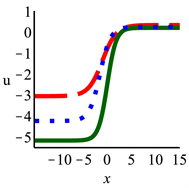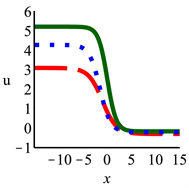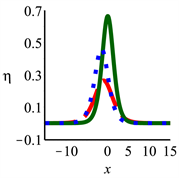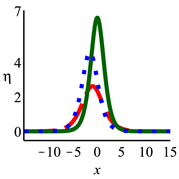Figure 2. The cross-section at t = 0 of the horizontal velocity $u\left(x,t\right)$ and height deviation $\eta \left(x,t\right)$ for solutions with ${R}_{s}>0$ (Group 1) and ${R}_{s}<0$ (Group 4), respectively. The left two panels are for case 1 and right two panels for case 2 with $\alpha =2$, $\beta =2$, $C=1/2$ and $k=1$. Solid line ( $A=3,q=1$ ), dashed line ( $A=2,q=2$ ) and dotted line ( $A=5/2,q=4$ ) are shown here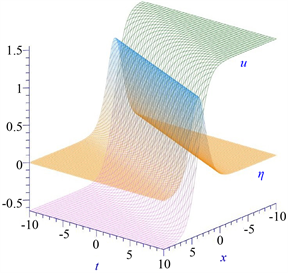(a)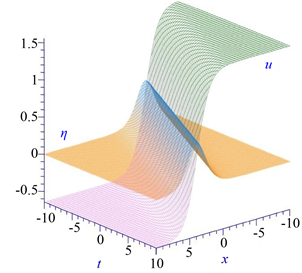(b)

Figure 3. The evolution behavior of $u\left(x,t\right)$ and $\eta \left(x,t\right)$ for classical long wave equation if $\alpha =0$ (a) and variant Boussinesq equation if $\alpha =1$ and $\beta =0$ (b)

5. 总结

NOTES

*第一作者。

#通讯作者。

  Debnath, L. (2012) Nonlinear Partial Differential Equations for Scientists and Engineers. 3rd Edition, Birkhäuser, Boston. https://doi.org/10.1007/978-0-8176-8265-1  Arendt, W., Brezis, H. and Pierre, M. (2004) Nonlinear Evolution Equations and Related Topics. Birkhäuser, Basel. https://doi.org/10.1007/978-3-0348-7924-8  Pelinovsky, E. and Kharif, C. (Eds.) (2016) Extreme Ocean Waves. Springer, Cham, 12-15. https://doi.org/10.1007/978-3-319-21575-4  Lin, C. and Clark, J. (1959) On the Theory of Shallow Water Waves. Tsing Hua Journal of Chinese Studies, 1, 54-62.  Whitham, G. (1967) Variational Methods and Applica-tions to Water Waves. Proceedings of the Royal Society of London. Series A. Mathematical and Physical Sciences, 299, 6-25. https://doi.org/10.1098/rspa.1967.0119  Broer, L. (1975) Approximate Equations for Long Water Waves. Applied Scientific Research, 31, 377-395. https://doi.org/10.1007/BF00418048  Kaup, D. (1975) A Higher-Order Water-Wave Equation and the Method for Solving It. Progress of Theoretical Physics, 54, 396-408. https://doi.org/10.1143/PTP.54.396  Zhang, Z.Y., Yong, X.L. and Chen, Y.F. (2008) Symmetry Analysis for Whitham-Broer-Kaup Equations. Journal of Nonlinear Mathematical Physics, 15, 383-397. https://doi.org/10.2991/jnmp.2008.15.4.3  Xu, T.T. (2015) Darboux Transformation and New Multi-Soliton Solutions of the Whitham-Broer-Kaup System. Applied Mathematics, 6, 20-27. https://doi.org/10.4236/am.2015.61003  Fan, E.G. and Zhang, H.Q. (1998) Backlund Transformation and Ex-act Solutions for Whitham-Broer-Kaup Equations in Shallow Water. Applied Mathematics and Mechanics, 19, 713-716. https://doi.org/10.1007/BF02457745  Zheng, Z. and Shan, W.R. (2009) Application of Exp-Function Method to the Whitham-Broer-Kaup Shallow Water Model Using Symbolic Computation. Applied Mathematics and Computation, 215, 2390-2396. https://doi.org/10.1016/j.amc.2009.08.032  Lou, S.Y. (2015) Consistent Riccati Expansion for Integrable Sys-tems. Studies in Applied Mathematics, 134, 372-402. https://doi.org/10.1111/sapm.12072